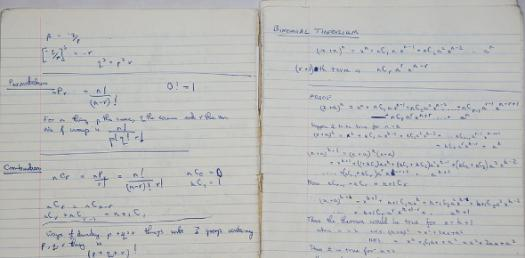# An Interesting Quiz On Binomial Theorem

14 Questions | Attempts: 545
ShareSettingsWhat do you know of binomial theorem? It’s a formula for finding any power of a binomial without multiplying at length. It’s a mathematical theorem that gives the expansion of any binomial raised to a positive integral power. Binomial Theorem Quiz will help you understand how binomial theorem is used mathematically and also in real life situation. Good luck.

• 1.
Find the 7th term of (2+x)^10.
• A.

3360x^6

• B.

3000x^6

• C.

4500x^7

• 2.
Find the coeeficient of x in the expansion of (x-3/x)^9.
• A.

10500

• B.

10206

• C.

12100

• 3.
Find the coefficient of x^6 in (1-3x)^8
• A.

20412

• B.

10512

• C.

23110

• 4.
Obtain in descending powers of x the first four terms in the expansion of (2x-1)^6.
• 5.
In the expansion of (x^3-2/x^2)^10, find the coefficient of 1/x^5.
• A.

13440

• B.

3360

• C.

-15360

• 6.
Find the coefficient of x^3 in the expansion of (3-4x)(2-x/2)^7
• A.

435

• B.

-882

• C.

632

• 7.
Who created the special triangle?
• 8.
In the expansion of (2+3x)^n, the coefficients of x^3 and x^4 are in the ratio of 8:15. Find the value of n.
• A.

10

• B.

8

• C.

3

• 9.
The binomial expansion of (1-b)^  is the same as that of (1+b)^n except that the coefficients have alternate + and - signs.
• A.

True

• B.

False

• 10.
Find the coefficients of x^2 in the expansion of (1-2x)^5.
• A.

23

• B.

40

• C.

51

• 11.
There is no difference between the power n and the expansion of the binomial theorem.
• A.

True

• B.

False

• 12.
Find the term x^3 in the expansion of (1+5)^2(1-2x)^6.
• A.

150x^3

• B.

140x^3

• C.

91x^3

• 13.
With the help of this binomial theorem for positive integral index indices , we can expand any power of x + y into a sum of terms forming a polynomial.
• A.

True

• B.

False

• 14.
BINOMIAL , in mathematics, a word first introduced by Robert Recorde (1557) to denote a quantity composed of the sum or difference to two terms; as a+b, a-b.
• A.

True

• B.

False

## Related TopicsBack to top
×

Wait!
Here's an interesting quiz for you.